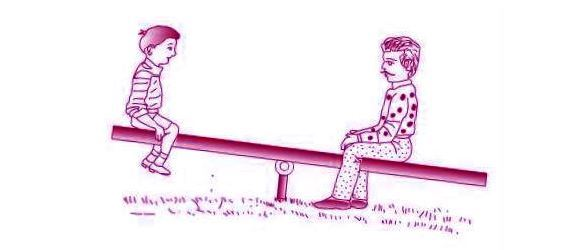# Moment Formula

## Momentum

Moment refers to a very short period of time. If you consider a see-saw, putting weights on both sides makes it to be in a balanced moment. If you put extra weight or less weight, on one hand, the see-saw is no more balanced, and this is known as the unbalanced moment.The measure of turning effect is known as torque. The force which acts on the body of the torque is known as moment of force.

The moment formula is given by

Moment of force = F x d

Where,

F is the force applied,

d is the distance from the fixed axis,

Moment of force is expressed in newton meter (Nm).

Moment of force formula can be applied to calculate the moment of force for balanced as well as unbalanced forces.

### Solved Examples

Example 1

A 200 cm meter rule is pivoted at the middle point (at 50 cm point). If the weight of 10 N is hanged from the 30 cm mark and a weight of 20 N is hanged from its 60 cm mark, identify whether the meter rule will remain balanced over its pivot or not.

Solution:

According to the principle of moments, when an object is in rotational equilibrium, then

Total anticlockwise moments = Total clockwise moments

Total anticlockwise moments:

Length of lever arm = (50 – 30) = 20 cm

= 0.20 m

Since the length of the lever arm is the distance from its mid-point, where its balanced force applied = 10 N

Anticlockwise moment = Lever arm x Force applied

= 0.20 x 10 = 2 Nm

Clockwise moment: Length of lever arm = (60 – 50)

= 10 cm

= 0.10 m.

Since the length of the lever arm is the distance from the mid-point, about which balanced Force applied = 20 N

Clockwise moment = lever arm x force applied

= 0.10 × 20 = 2 Nm

Therefore,

Since the total anti-clockwise moment = total clockwise moment = 2 Nm, according to the principle of moments, it is in rotational equilibrium ie, the meter rule remains balanced about its pivot.

Example 2

A 500 cm meter rule is pivoted at its middle point. If weight of 2 N is hanged from the 20 cm point, Calculate the amount of weight required to be applied at the 80 cm mark to keep it in a balanced position.

Solution:

According to the principle of moments, To keep an object in rotational equilibrium, the sum of anticlockwise moments and clockwise moments acting should be equal. Therefore, the amount of weight to be hanged from the 80 cm mark must be able to generate a clockwise moment equal to the anticlockwise moment generated by the weight hanged on the left side of the meter rule.

Anticlockwise moment :

Length of lever arm = (50 – 20)

= 30 cm

= 0.30 m

Since the length of the lever arm is the distance from its mid-point, where it is balanced

Force applied = 2 N

Anticlockwise moment = lever arm x force applied

= 0.30 x 2 N

= 0.6 Nm

Clockwise moment:

length of lever arm = (80 – 50)

= 30 cm

= 0.30 m

Since the length of the lever arm is the distance from its Force applied.

Let it be ‘F’.

Thus, clockwise moment = F x 0.30

= 0.30 F Nm

Clockwise moment = Anticlockwise moment

0.30 F = 0.6

F = 2 N

A weight of 2 N needs to be hanged from  80 cm point to keep the meter rule balanced.

Stay tuned with BYJU’S for more such interesting articles. Also, register to “BYJU’S – The Learning App” for loads of interactive, engaging Physics-related videos and an unlimited academic assist.